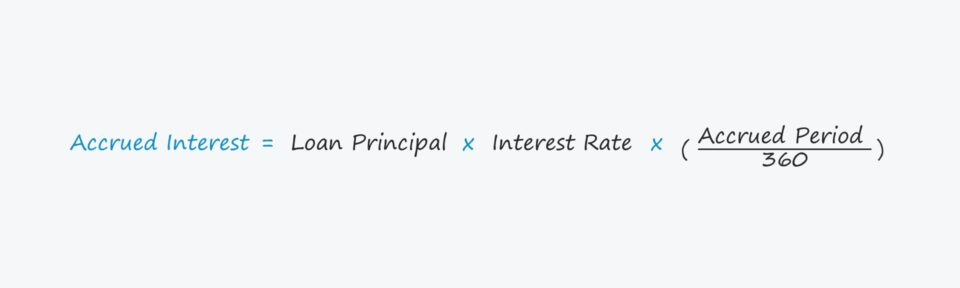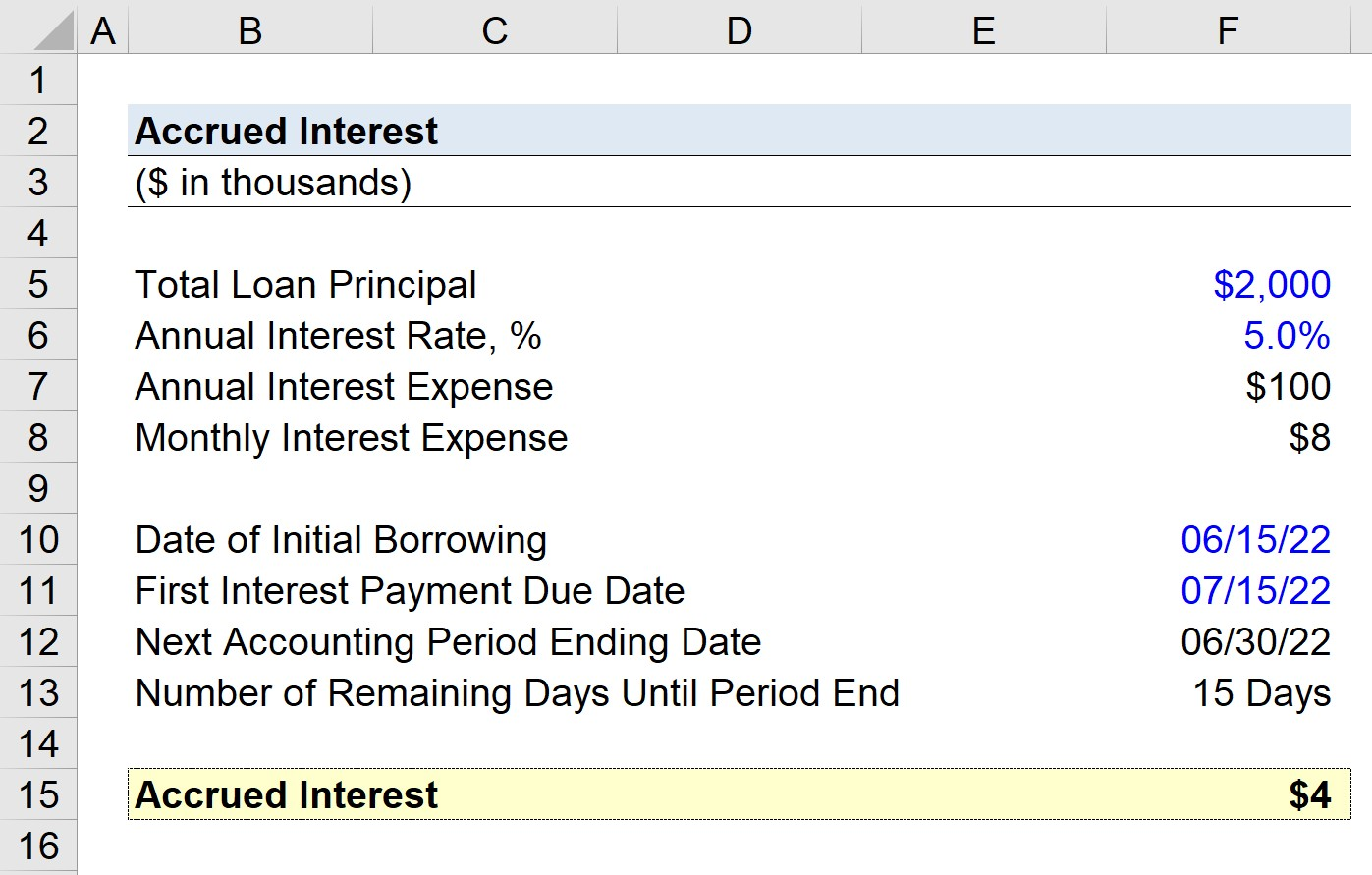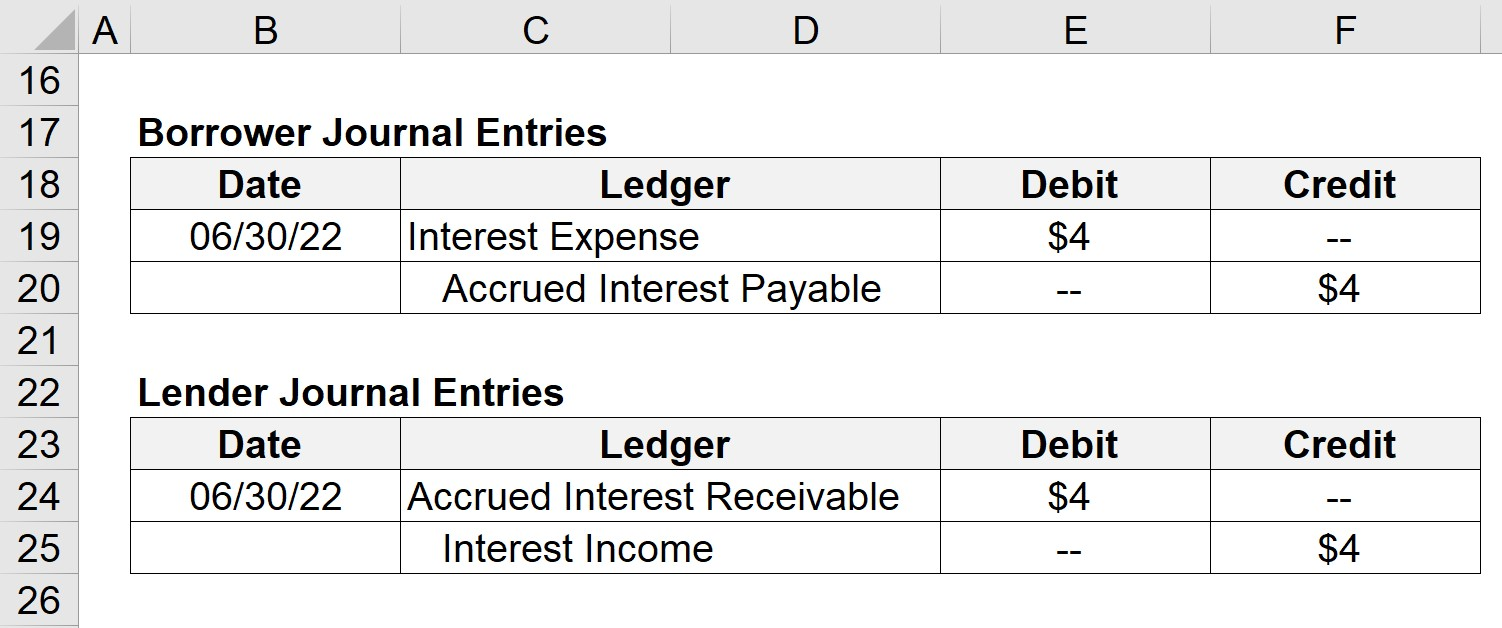# Accrued Interest

Guide to Understanding Accrued Interest## How to Calculate Accrued Interest (Step-by-Step)

The term “accrued interest” refers to the total interest owed to a lender on a specified date.

Most debt financing arrangements, such as loans, require the borrower to make periodic interest payments to the lender in exchange for capital.

But in the case here, the borrower has not yet paid the lender (and the lender has not yet received the owed interest payment).

Per GAAP accounting reporting standards, all transactions must be recorded in the “correct” period, in an effort to ensure consistency and transparency for investors.

Specifically, transactions under accrual accounting must be recorded on the date of occurrence (i.e. once earned), irrespective of whether cash payment was received.

## Accrued Interest Accounting: Journal Entry (Debit and Credit)

Under accrual accounting reporting standards established by GAAP, any interest that accrued is required to be recorded along with an accrual, i.e. an adjusting entry to reflect that the interest remains unpaid.

• Borrower Journal Entry: On the ledger of the borrower, the adjusting entries are a debit to the “Interest Expense” account and a credit to the “Accrued Interest Payable” account.
• Lender Journal Entry: In contrast, the lender would debit the “Accrued Interest Receivable” account and credit the “Interest Income” account.

The accrued interest payable is recognized as a current liability, whereas the receivable counterpart is recorded as a current asset since both are assumed to be resolved soon (<12 months).

Once the interest amount is paid in cash, the journal entries will be adjusted to reflect that the borrower has paid the owed interest to the lender.

## Accrued Interest Formula

The formula for calculating accrued interest is as follows.

Accrued Interest = Loan Principal * [Interest Rate x (Days / 360)]
• Loan Principal: The original loan amount on the date of initial issuance.
• Interest Rate (%): The cost of financing charged by the lender on the loan.
• Days: The number of days until the end of the month.

## Accrued Interest Calculator – Excel Model Template

We’ll now move to a modeling exercise, which you can access by filling out the form below.Submitting ...

## Step 1. Loan Financing and Interest Rate Assumptions

Suppose a company has raised \$2 million in debt financing on June 15, 2022, roughly the mid-point of the month.

The annual interest rate on the loan is 5%, which can be multiplied by the total loan amount to arrive at an annual interest expense of \$100k.

• Total Loan Principal = \$2mm
• Interest Rate = 5%
• Annual Interest Expense = \$2mm * 5% = \$100k

By dividing the annual interest expense by the number of months in a year (12) we can calculate the monthly interest expense as approximately \$8k.

• Monthly Interest Expense = \$100k / 12 = \$8k

Per the loan agreement, the first interest payment comes due in 30 days, i.e. July 15, 2022.

## Step 2. Accrued Interest Calculation Example

The monthly accounting period ends on June 30, 2022, meaning that there are 15 days remaining from the date of initial financing to the end of the month.

• Step 1: The amount of interest recognized as accrued can be calculated by first dividing the number of days until the end of the month by the number of days in the year (360 days).
• Step 2: In the next step, we’ll multiply the resulting figure from above by the annual interest rate (5%).
• Step 3: Finally, the resulting figure is multiplied by the total loan principal (\$2mm) to arrive at \$4k as the estimated amount, i.e. \$2mm * [5%*(15/360)] = \$4k## Step 3. Journal Entry Example (Debit and Credit)

As the end of the accounting period comes near, the borrower and lender must adjust their ledger to account for the interest that accrued.

Starting with the borrower, we’ll go through the journal entries in their ledger as of June 30, 2022.

• Interest Expense = \$4k Debit
• Accrued Interest Payable = \$4k Credit

In short, the adjustments above reflect how the interest was not yet paid, which is why the “Interest Expense” account was debited, and the “Accrued Interest Payable” account was credited.

On the other hand, the lender’s journal entries will be as follows.

The lender’s adjusting entry debited “Accrued Interest Receivable” and credited “Interest Income”.

Once the next accounting period rolls around, these adjusting entries would be reversed.Step-by-Step Online Course

### Everything You Need To Master Financial Modeling

Enroll in The Premium Package: Learn Financial Statement Modeling, DCF, M&A, LBO and Comps. The same training program used at top investment banks.